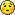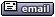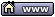flat assembler
Message board for the users of flat assembler.flat assembler > DOS > convert mantissa bits to decimal fraction number
Author
 Threadmns

Joined: 20 Dec 2007
Posts: 101
Location: Piliyandala,Sri lanka
I'm trying to convert ieee single precision number generated by fpu to decimal number with assembly(without c library).I could convert sign and exponent bits.but struggling to convert mantissa bits to decimal fraction number(with a assembly routine).as number generating on the process cannot put to cpu register as it is larger than register. Please help?
for example (ieee single precision number)
0 01111111 01011110101110000101001
how to get the decimal number of 01011110101110000101001 (which is 0.36921875476837158203125) using assembly ?[/i]04 Jun 2015, 19:02smiddy

Joined: 31 Oct 2004
Posts: 559
I have yet to crack that nut, since I am not working on it yet. I will when I write the calculator for my OS. Take a look at this and see if this gives you some ideas: http://www.x86asm.net/articles/working-with-big-numbers-using-x86-instructions/05 Jun 2015, 02:53mns

Joined: 20 Dec 2007
Posts: 101
Location: Piliyandala,Sri lanka
thanks smiddy.i think it will give a idea for the problem(though i have lot to understand)

Last edited by mns on 05 Jun 2015, 11:56; edited 1 time in total05 Jun 2015, 05:56revolution
When all else fails, read the source

Joined: 24 Aug 2004
Posts: 16628
The basic idea is to multiply by some power of 10 to get the required number of digits precision.

For example your binary number 01011110101110000101001 (which is 0.37000000476837158203125) can be converted to 4 digits of precision by multiplying by 10^4 and dividing by 2^23.

(0x2F5C29 * 10000) shr 23 = 3700.05 Jun 2015, 06:10mns

Joined: 20 Dec 2007
Posts: 101
Location: Piliyandala,Sri lanka
thank you revolution.but i want to convert all 23 bits of mantissa field to decimal number and display it(using assembly code).i think my actual problem is, dealing with large numbers in assembly.05 Jun 2015, 12:45revolution
When all else fails, read the source

Joined: 24 Aug 2004
Posts: 16628
23 bits doesn't need any special coding. If you want a full 7 digits precision then you can do this:
Code:
```mov eax,0x2F5C29 shl (32-23) ;move to high order bits
mov edx,10000000
mul edx ;edx = 3700000    ```05 Jun 2015, 12:51revolution
When all else fails, read the source

Joined: 24 Aug 2004
Posts: 16628
I should probably add that you can keep extracting more more digits by continuing the code:
Code:
```mov eax,0x2F5C29 shl (32-23) ;move to high order bits
mov ecx,1000000000      ;get 9 digits per pass
mul ecx ;edx = 370000004
;<print first 9 digits>
mul ecx ;edx = 768371582
;<print next 9 digits>
mul ecx ;edx = 031250000
;<print next 9 digits>
etc.    ```
This works because we are implicitly assuming the unstored bits to the right of the mantissa are all zeros. But also note that this is extracting more precision than is present in the original number.05 Jun 2015, 14:34mns

Joined: 20 Dec 2007
Posts: 101
Location: Piliyandala,Sri lanka
thank you revolution.your solution works fine.but still i'm trying to figure out how it works and what is the math behind it.I'm grateful if you can explain more.05 Jun 2015, 19:26revolution
When all else fails, read the source

Joined: 24 Aug 2004
Posts: 16628
The premise is that the binary number is implied to be 0.xxxx... That is, the number is [0,1). Only 23-bits are given but it is assumed that other trailing bits are all zeros.

After each mul instruction the values to the left of the binary point are printed and then discarded, and the values to the right of the binary point are kept for the next iteration. After mul it looks like this: yyyy.xxxx... Then yyyy is printed and the remaining 0.xxxx... is run through again.06 Jun 2015, 15:30mns

Joined: 20 Dec 2007
Posts: 101
Location: Piliyandala,Sri lanka
thanks.but how these bits interpreted as fraction numbers(0.xxxx) and not integers(xxxx) with your code.in other words, what is the mathematical equation your code based on?
sorry for bothering you so much.(i think i have a long way to go to understand even basic things in assembly).06 Jun 2015, 18:44revolution
When all else fails, read the source

Joined: 24 Aug 2004
Posts: 16628
EAX contains the xxxx part and the binary point is at bit 32. After the MUL instruction EDX contains the yyyy part and EAX contains the next xxxx part. This is essentially a fixed point representation of 32.32.07 Jun 2015, 01:19mns

Joined: 20 Dec 2007
Posts: 101
Location: Piliyandala,Sri lanka
thanks again revolution.i got the idea.
it is like,
let's say binary fraction .1101
- if it is a complete number it would be (1101) = 13 in decimal
-as it is a binary fraction it is = 13/16 in decimal
which is 13/2^4
which is (1x2^(4-1))+(1x2^(4-2))+(0x2^(4-3))+(1x2^(4-4))/2^4

like that
.01011110101110000101001 in decimal is,
((1or0)x2^(23-1))+((1or0)x2^(23-2))+((1or0)x2^(23-3))+((1or0)x2^(23-4))+........./2^23

when shl (32-23) it will be,
((1or0)x2^(32-1))+((1or0)x2^(32-2))+((1or0)x2^(32-3))+((1or0)x2^(32-4))+........./2^32

when mul, actual division by 2^32 take place and and the result will be at edx.(i hope i'm correct in this )07 Jun 2015, 04:46El Tangas

Joined: 11 Oct 2003
Posts: 120
Location: Sunset Empire
Yes, that's it. If you want to convert it to a string for printing, you can multiply just by 10 so that you only extract one decimal digit at a time. The remainder will be left in EAX, repeat until EAX is zero (or until you are satisfied with the decimal precision for display). EAX will always eventually reach zero, because any finite lenght binary fraction can be represented by a finite lenght decimal fraction (in fact they will have the same number of digits). Can take a while, though...
For printing, add each result in EDX to '0' char and build the string.07 Jun 2015, 23:36mns

Joined: 20 Dec 2007
Posts: 101
Location: Piliyandala,Sri lanka
good,thank you very much El Tangas and revolution for you kind help.08 Jun 2015, 03:55El Tangas

Joined: 11 Oct 2003
Posts: 120
Location: Sunset Empire
Just one more thing, in case you want to convert an ieee float to decimal to print in scientific notation, some adjustments have to be made to the binary mantissa and exponent beforehand.

1) Exponent
multiply the binary exponent by the constant log_10(2) that you can for exemple load in the x87 fpu with the fldlg2 instruction. This is an important constant for these conversions, that's why it's hardcoded in the fpu. Take the integer part of the result as the decimal exponent. Then calculate 10^(remainder) for the next step.

2) Mantissa
Take the binary mantissa and multiply by 10^(remainder) calculated before, to obtain the decimal mantissa.

You will notice (using log and exponent properties) that these operations actually don't change the number, just adjust from M*2^a to N*10^b forms.

Once you have the adjusted exponent and mantissa, you can convert to decimal using the techniques shown before.08 Jun 2015, 09:50revolution
When all else fails, read the source

Joined: 24 Aug 2004
Posts: 16628
El Tangas wrote:
Just one more thing, in case you want to convert an ieee float to decimal to print in scientific notation, some adjustments have to be made to the binary mantissa and exponent beforehand.

1) Exponent
multiply the binary exponent by the constant log_10(2) that you can for exemple load in the x87 fpu with the fldlg2 instruction. This is an important constant for these conversions, that's why it's hardcoded in the fpu. Take the integer part of the result as the decimal exponent. Then calculate 10^(remainder) for the next step.

2) Mantissa
Take the binary mantissa and multiply by 10^(remainder) calculated before, to obtain the decimal mantissa.

You will notice (using log and exponent properties) that these operations actually don't change the number, just adjust from M*2^a to N*10^b forms.

Once you have the adjusted exponent and mantissa, you can convert to decimal using the techniques shown before.
This will work okay if one of not concerned about perfect accuracy of conversion. And it is also tricky to arrange the operations just right to get the most number of accurate digits. Depending upon your goals this would be fine for most circumstances, but if not then other techniques are needed to obtain complete accuracy for all possible digits.08 Jun 2015, 11:26redsock

Joined: 09 Oct 2009
Posts: 306
Location: Australia
FWIW, this is a good read, and how I implemented it in my own library: http://www.cs.indiana.edu/~dyb/pubs/FP-Printing-PLDI96.pdf08 Jun 2015, 22:16mns

Joined: 20 Dec 2007
Posts: 101
Location: Piliyandala,Sri lanka
again thank you all for your invaluable replies.10 Jun 2015, 11:36Display posts from previous: All Posts1 Day7 Days2 Weeks1 Month3 Months6 Months1 Year Oldest FirstNewest FirstJump to: Select a forum Official----------------AssemblyPeripheria General----------------MainDOSWindowsLinuxUnixMenuetOS Specific----------------MacroinstructionsCompiler InternalsIDE DevelopmentOS ConstructionNon-x86 architecturesHigh Level LanguagesProgramming Language DesignProjects and IdeasExamples and Tutorials Other----------------FeedbackHeapTest Area

Forum Rules:
 You cannot post new topics in this forumYou cannot reply to topics in this forumYou cannot edit your posts in this forumYou cannot delete your posts in this forumYou cannot vote in polls in this forumYou cannot attach files in this forumYou can download files in this forum